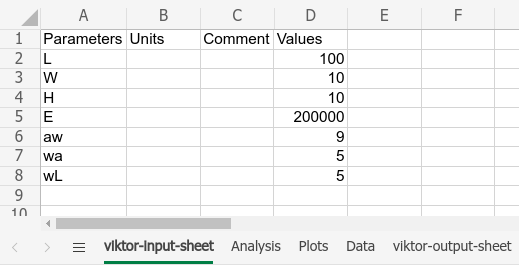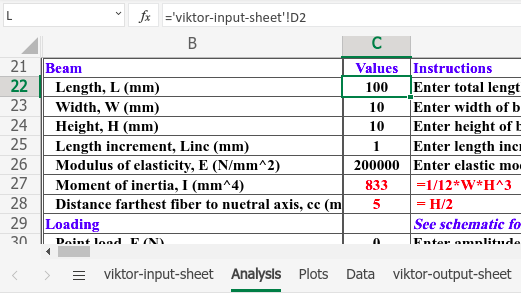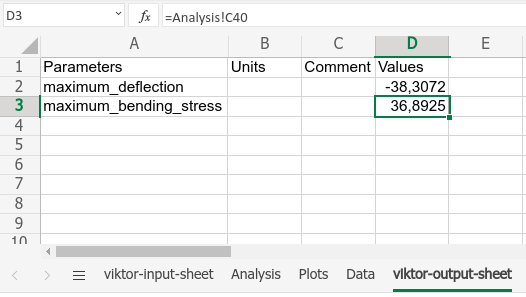# Tutorial - Using a calculation spreadsheet

note

Estimated time: 30 minutes

## Introduction​

Welcome to the spreadsheet calculation tutorial! In construction engineering (and many other branches of engineering for that matter) knowledge is made explicit using Excel sheets.

In this tutorial you will learn how to implement an existing calculations from an Excel sheet into a VIKTOR app. The example case we'll use is to create an application to analyze a simply supported beam under load. A spreadsheet will be used for the calculations.

Download the starting app to your hard disk. Run the `install` command from within the app folder to install the app and its dependencies, `clear` any previous saved local database, and run `start` to start to app:

``viktor-cli installviktor-cli clearviktor-cli start``

In the browser, click the "Example" entity to open its editor and verify the app is installed and running as expected.

## Folder structure​

The app has the following folder structure:

``spreadsheet-tutorial├── app.py├── beam_calculation.xls ├── beam_schematic.svg├── requirements.txt└── viktor.config.toml``

 source: https://stuff.mit.edu (04-05-2020)

Open `app.py` and have a look at the code that we have set up for you. Note that the code already has some basic class structure. We will extend the code, step-by-step, throughout this tutorial.

To be able to use a spreadsheet as a calculation tool within a VIKTOR app, a few modifications must be performed to the spreadsheet file. Open `beam_calculation.xls` and add two sheets: `viktor-input-sheet` and `viktor-output-sheet`. These sheets contain the input and output values respectively. In the `viktor-input-sheet`, add the following data:The values from `viktor-input-sheet` should now be used in the `Analysis` sheet. For example, cell C22 below refers to the "L" parameter defined in the `viktor-input-sheet` sheet:In the `viktor-output-sheet` the desired output parameters are defined, with their values taken from the `Analysis` sheet:You should now be able to change the values on the `viktor-input-sheet`, resulting in changed values in the `viktor-output-sheet`.

The spreadsheet is now ready for use within the app. VIKTOR provides a helper class specifically for this task: `SpreadsheetCalculation`. Open `app.py` and replace the method `get_data_view` with the following code (mind the extra imports):

``from viktor.external.spreadsheet import SpreadsheetCalculationInput, SpreadsheetCalculationclass Calculation(ViktorController):    ...    @DataView('Results', duration_guess=1)    def get_data_view(self, params, **kwargs):        inputs = [            SpreadsheetCalculationInput('L', params.beam.length),            SpreadsheetCalculationInput('W', params.beam.width),            SpreadsheetCalculationInput('H', params.beam.height),            SpreadsheetCalculationInput('E', params.beam.E),            SpreadsheetCalculationInput('aw', params.loads.aw),            SpreadsheetCalculationInput('wa', params.loads.wa),            SpreadsheetCalculationInput('wL', params.loads.wL),        ]        spreadsheet = File.from_path(Path(__file__).parent / 'beam_calculation.xls')        sheet = SpreadsheetCalculation(spreadsheet, inputs=inputs)        result = sheet.evaluate(include_filled_file=True)        print(result.values)        data = DataGroup(            maximum_deflection=DataItem('Maximum deflection', -10, suffix='microns', number_of_decimals=2),            maximum_bending_stress=DataItem('Maximum bending stress', -13, suffix='N/mm2', number_of_decimals=2),        )        return DataResult(data)``

The input parameters are accessed from the params dictionary and follow the same structure as defined in the parametrization class.

In your browser change the value of `Length` from `100` to `120` and notice that the updated values are now printed to the command-line.

## Showing the output​

To display the output in the app's interface, the parameters from the `result` dictionary must be linked to the `DataItem`s, as shown below. Modify the method `get_data_view` as follows:

``    def get_data_view(self, params, **kwargs):        ...        data = DataGroup(            maximum_deflection=DataItem('Maximum deflection', result.values['maximum_deflection'], suffix='microns', number_of_decimals=2),            maximum_bending_stress=DataItem('Maximum bending stress', result.values['maximum_bending_stress'], suffix='N/mm2', number_of_decimals=2),        )        ...``

Confirm that changing the value in your browser gives you different outputs in the `DataView`.

A nice addition to the app would be that the sheet, used for the calculation, can be downloaded. Add a `DownloadButton` to the parametrization (mind the extra import):

``from viktor.parametrization import ..., DownloadButtonclass Parametrization(ViktorParametrization):    ...    downloads = Section('Downloads')    downloads.spreadsheet = DownloadButton('Download', 'download_spreadsheet')``

The `download_spreadsheet` method should contain the downloading logic and must be added in the `Calculation` class. To prevent writing duplicate code, however, we will first restructure this code a bit. Let's create a new method called `get_evaluated_spreadsheet`, which will be called by both `get_data_view` and `download_spreadsheet`:

``class Calculation(ViktorController):    ...    def get_evaluated_spreadsheet(self, params):        inputs = [            SpreadsheetCalculationInput('L', params.beam.length),            SpreadsheetCalculationInput('W', params.beam.width),            SpreadsheetCalculationInput('H', params.beam.height),            SpreadsheetCalculationInput('E', params.beam.E),            SpreadsheetCalculationInput('aw', params.loads.aw),            SpreadsheetCalculationInput('wa', params.loads.wa),            SpreadsheetCalculationInput('wL', params.loads.wL),        ]        spreadsheet = File.from_path(Path(__file__).parent / 'beam_calculation.xls')        sheet = SpreadsheetCalculation(spreadsheet, inputs=inputs)        result = sheet.evaluate(include_filled_file=True)        return result    @DataView('Results', duration_guess=1)    def get_data_view(self, params, **kwargs):        result = self.get_evaluated_spreadsheet(params)        max_deflection = result.values['maximum_deflection']        max_bending_stress = result.values['maximum_bending_stress']        data = DataGroup(            maximum_deflection=DataItem('Maximum deflection', max_deflection, suffix='microns', number_of_decimals=2),            maximum_bending_stress=DataItem('Maximum bending stress', max_bending_stress, suffix='N/mm2', number_of_decimals=2),        )        return DataResult(data)``

Now we add the method `download_spreadsheet`, which uses this newly created method (mind the extra import):

``...from viktor.result import DownloadResultclass Calculation(ViktorController):    ...    def download_spreadsheet(self, params, **kwargs):        result = self.get_evaluated_spreadsheet(params)        return DownloadResult(result.file_content, 'evaluated_beam.xlsx')``